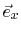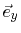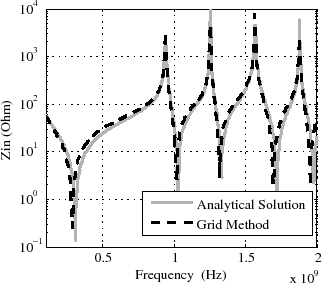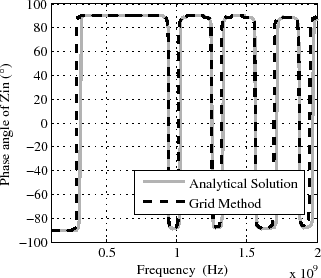# 4.3 Inductance, capacitance, resistance (LCR) grid solution method for the two-dimensional Helmholtz equation

Through cartesian discretization of the differential operator in (4.13),  obtained a finite difference method on a rectangular grid. With interpretation according to the TLM method , , a method with lumped inductances, capacitances, resistances and admittances on a rectangular mesh was presented by  and . The value for the lumped resistance is obtained from (4.11) and that for the lumped admittance from (4.12). The value of the lumped inductance is calculated directly from (4.9), while the lumped capacitance is obtained by multiplication of the capacitance from (4.9) with DxDy. Dx and Dy denote the rectangular grid cell lengths in x-direction and in y-direction, respectively. Other circuit elements can be introduced easily at every point of the grid. The circuit structure of the lumped elements on the grid leads to a sparse matrix. However, a very fine grid has to be used to obtain reasonably good accuracy. Figure 4.4 depicts a comparison of the analytic results from (4.18) to the LCR grid method results for the impedance Zin of a port at position (x=10mm, y=10mm) on a rectangular cavity with dimensions (L=160mm, W=120mm) and h=7mm. A grid spaciny of Dx = Dy = 2mm was used for the LCR grid method simulation. Even with that fine grid the comparison shows some slight deviations of the impedance magnitude minima, which indicate a small inaccuracy of simulated inductance. This is a disadvantage of the method, because the necessity of the fine grid leads to higher simulation costs compared to the FEM method in Section 4.4.
Rectangular planes have been used for the comparison in Figure 4.4 to enable the comparison with the analytical solution. When the method is used for planes with edges that are not parallel to one of the cartesian directionsor, a dense grid will be necessary to obtain an accurate discretization of the geometry at that edge. Although sub-gridding at the edge is an opportunity to reduce the overall mesh size, the effort for geometry discretization is much higher compared to the finite element method in Section 4.4, which uses triangular meshing.(a) Magnitude comparison. (b) Phase angle comparison.
Figure 4.4: Comparison of Zin between the analytical solution (4.18) and the LCR grid method solution. Cavity dimensions are (L=160mm, W=120mm, h=7mm), the position of the port for the Zin is (x=10mm, y=10mm) and the grid spacing is Dx = Dy = 2mm.

C. Poschalko: The Simulation of Emission from Printed Circuit Boards under a Metallic Cover